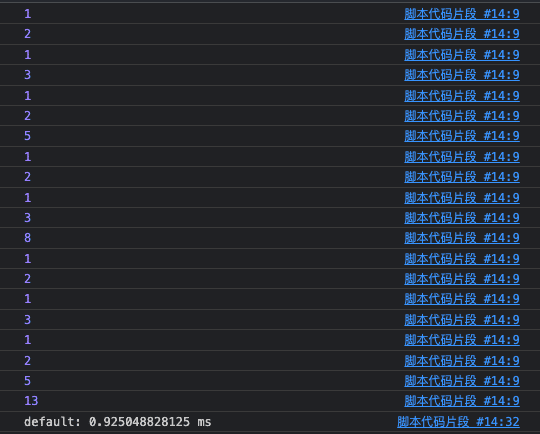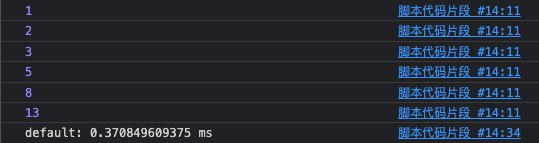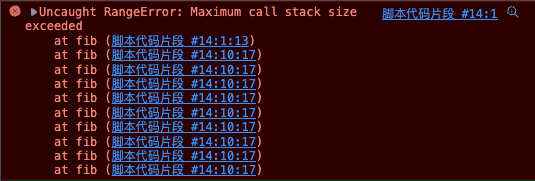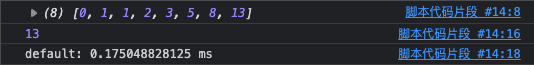# 一位未曾涉足算法的初学者收获

· 阅读需 10 分钟

## 那我为何会接触算法呢？​

``function fib(n) {  if (n <= 1) return n  return fib(n - 1) + fib(n - 2)}``

### 对上述代码进行优化​

``function fib(n) {  if (n <= 1) {    return n  }  const res = fib(n - 1) + fib(n - 2)  console.log(res)  return res}````function fib(n, mem = []) {  if (n <= 1) {    return n  }  if (mem[n]) {    return mem[n]  }  const res = fib(n - 1, mem) + fib(n - 2, mem)  console.log(res)  mem[n] = res  return res}````function fib(n) {  const dp = [0, 1]  for (let i = 2; i <= n; i++) {    dp[i] = dp[i - 1] + dp[i - 2]  }  return dp[n]}``## 两数之和​

### 暴力枚举​

``var twoSum = function (nums, target) {  const n = nums.length  for (let i = 0; i < n; i++) {    for (let j = 0; j < n; j++) {      if (nums[i] + nums[j] === target && i !== j) {        return [i, j]      }    }  }}``

### 哈希表​

``var twoSum = function (nums, target) {  const map = new Map()  for (let i = 0; i < nums.length; i++) {    if (map.has(target - nums[i])) {      return [map.get(target - nums[i]), i]    }    map.set(nums[i], i)  }}``

### 双指针算法(特殊情况)​

1. 定义两个坐标(指针)分别指向数组成员最左边与最右边，命名为 left 与 right。
2. 使用 while 循环，循环条件为 left < right。
3. 判断 `nums[left] + nums[right]``target` 的大小关系，如果相等则说明找到目标(答案)，如果大于则 右指针减 1 `right—-`，小于则左指针加 1 `left++`
``function twoSum(nums, target) {  let left = 0  let right = nums.length - 1  while (left < right) {    const sum = nums[left] + nums[right]    if (sum === target) {      return [left, right]    }    if (sum > target) {      right--    } else if (sum < target) {      left++    }  }}``

## 结语​

If you want to become a good programmer, you can spend 10 years programming, or spend 2 years programming and learning algorithms.